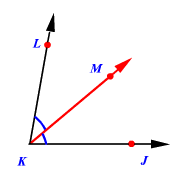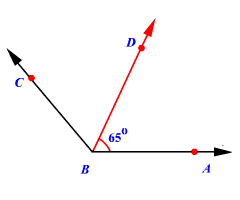# Angle Bisector

An angle bisector is a line or ray that divides an angle into two congruent angles .In the figure, the ray $\stackrel{\to }{KM}$ bisects the angle $\angle JKL$ .

The angles $\angle JKM$ and $\angle LKM$ are congruent.

So, $m\angle JKM=m\angle LKM$ .

Note that any point on the angle bisector is equidistant from the two sides of the angle. Some textbooks call this Angle Bisector Theorem , but this name is usually used for another theorem about angle bisectors in a triangle.

Example :In the figure, $\stackrel{\to }{BD}$ is an angle bisector. Find the measure of $\angle ABC$ .

Since $\stackrel{\to }{BD}$ is an angle bisector,

$m\angle CBD=m\angle ABD$

Therefore,

$m\angle CBD=65°$

$m\angle ABC=m\angle ABD+m\angle CBD$ .
$\begin{array}{l}m\angle ABC=65°+65°\\ \text{\hspace{0.17em}}\text{\hspace{0.17em}}\text{\hspace{0.17em}}\text{\hspace{0.17em}}\text{\hspace{0.17em}}\text{\hspace{0.17em}}\text{\hspace{0.17em}}\text{\hspace{0.17em}}\text{\hspace{0.17em}}\text{\hspace{0.17em}}\text{\hspace{0.17em}}\text{\hspace{0.17em}}\text{\hspace{0.17em}}\text{\hspace{0.17em}}\text{\hspace{0.17em}}\text{\hspace{0.17em}}\text{\hspace{0.17em}}\text{\hspace{0.17em}}\text{\hspace{0.17em}}\text{\hspace{0.17em}}=130°\end{array}$